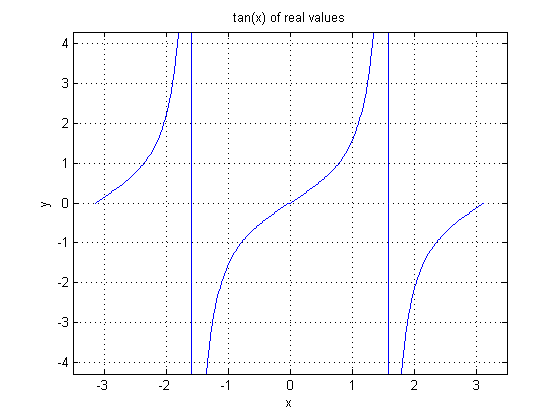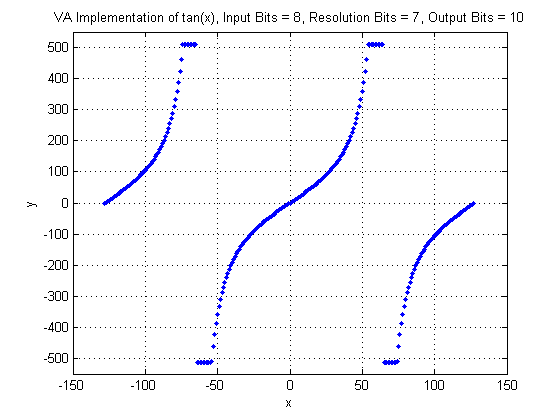## Operator TAN

The operator TAN calculates the tangent of the input.

The input range of the tangent function is [-∞, ∞]. Because of the periodicity of the tangent function the input range of the VisualApplets operator is limited to [-π, π[ i.e. the minimum value at the input is -π and the maximum value at the input plus 1 is π. Thus, it it not possible to have the value +π at the input.

The argument x of the tangent function is therefore determined by

where

is the bit width at the input link.

The results of the tangent function are in the range [-∞, ∞]. This operator includes a parameter ResolutionBits to define the fixed point resolution bits of the output values. The output range is therefore given by the number of output bits and the resolution bits =

where

is the bit width at the output and R the resolution bits. Thus, the output value is

The values -π/2 and π/2 are not defined for the tangent function. The VisualApplets operator will clip these values to the maximum or minimum possible values at the output. Moreover, the result of the tangent function can be out of the output value range. In this case, the results will be clipped to the maximum or minimum possible values, too. Thus,

The following image shows the plot of the normal tangent function.In the next figure, the VisualApplets operator implementation is shown. Note the input and output bit widths.### I/O Properties

Property Value
Operator Type O
Input Link I, data input
Output Link O, data output

### Supported Link Format

Bit Width [8, 12] [8,32]
Arithmetic signed signed
Parallelism any as I
Kernel Columns 1 as I
Kernel Rows 1 as I
Img Protocol {VALT_IMAGE2D, VALT_LINE1D, VALT_PIXEL0D} as I
Color Format VAF_GRAY as I
Color Flavor FL_NONE as I
Max. Img Width any as I
Max. Img Height any as I

### Parameters

ResolutionBits
Type static parameter
Default 8
Range [0, OutputBitWidth]

This parameter defines the accuracy of the input values as defined in the description above.